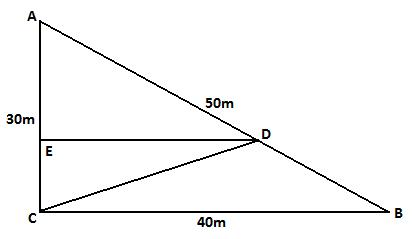#### You may also like### Some(?) of the Parts

A circle touches the lines OA, OB and AB where OA and OB are perpendicular. Show that the diameter of the circle is equal to the perimeter of the triangle### Coke Machine

The coke machine in college takes 50 pence pieces. It also takes a certain foreign coin of traditional design...### Contact

A circular plate rolls in contact with the sides of a rectangular tray. How much of its circumference comes into contact with the sides of the tray when it rolls around one circuit?

# Garden Fence

##### Age 14 to 16 Short Challenge Level:

In the diagram below, triangle $ABC$ represents the garden, $CD$ represents the fence and $E$ is the foot of the perpendicular from $D$ to $AC$.The two sections of the garden have the same perimeter so $AD$ is $10$m longer than $DB$. Hence $AD=30$m and $DB=20$m.

Triangles $AED$ and $ACB$ are similar so $\frac{AE}{AC}=\frac{AD}{AB}=\frac{30}{50}$. Hence $AE=\frac{3}{5}\times 30$m $=18$m. So $EC=(30-18)$m $=12$m.

Also $\frac{ED}{CB}=\frac{AD}{AB}=\frac{30}{50}$. Hence $ED=\frac{3}{5}\times 40$m $=24$m.

Finally, by Pythagoras: $CD^2=EC^2+ED^2=(12^2+24^2)$m$^2=5\times12^2$m$^2$. So the length of the fence is $12\sqrt{5}$m.
This problem is taken from the UKMT Mathematical Challenges.
You can find more short problems, arranged by curriculum topic, in our short problems collection.